Worksheets and No Prep Teaching Resources
Math Worksheets
Measurement Worksheets

# LengthWorksheets, Lessons, and Printables

Measuring Length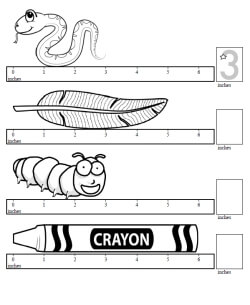Measure length in inches - first answer given Measure length in centimeters - first answer given

Measuring Length of ObjectsMeasure length in inches Measure length in centimeters

Sorting Length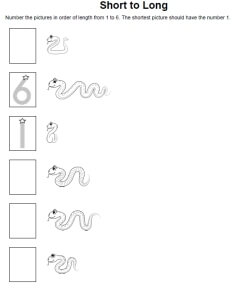Measure length in inches - 1 is shortest, 6 is longest (first and last answers given) Measure length in inches - 1 is longest, 6 is shortest (first and last answers given) Measure length in inches - mix (first and last answers given)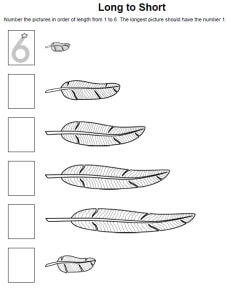Measure length in inches - 1 is shortest, 6 is longest (first answer given) Measure length in inches - 1 is longest, 6 is shortest (first answer given) Measure length in inches - mix (first answer given)

Sorting Length: One Answer Given (can be any number from 1 to 6)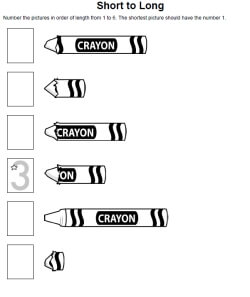Measure length in inches - 1 is shortest, 6 is longest (one answer given) Measure length in inches - 1 is longest, 6 is shortest (one answer given) Measure length in inches - mix (one answer given)

Length of Lines

Inches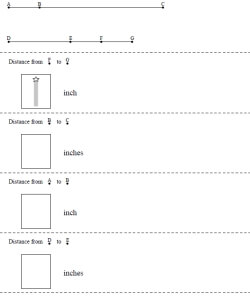Measure length in inches between points

Centimeters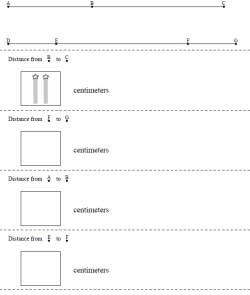Measure length in centimeters between points

Length of Boxes
Easier: Always the Same Direction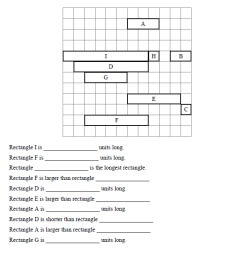Length: One large problem (4 to 6 rectangles) Length: One large problem (7 to 10 rectangles) Length: One large problem (11 to 15 rectangles)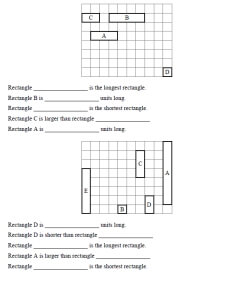Length: Two problems per page (4 to 6 rectangles) Length: Two problems per page (7 to 10 rectangles)

Different Directions on Same Problem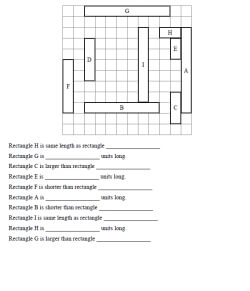Length: One large problem (4 to 6 rectangles) Length: One large problem (7 to 10 rectangles) Length: One large problem (11 to 15 rectangles)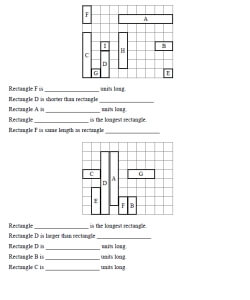Length: Two problems per page (4 to 6 rectangles) Length: Two problems per page (7 to 10 rectangles)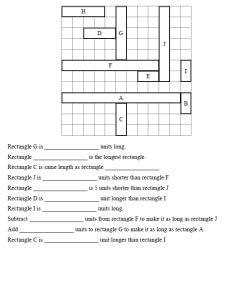Length with Addition and Subtraction Questions: One large problem (4 to 6 rectangles) Length with Addition and Subtraction Questions: One large problem (7 to 10 rectangles) Length with Addition and Subtraction Questions: One large problem (11 to 15 rectangles)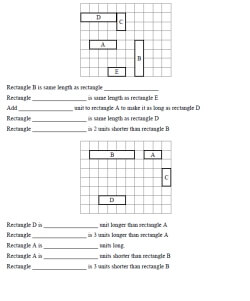Length with Addition and Subtraction Questions: Two problems per page (4 to 6 rectangles) Length with Addition and Subtraction Questions: Two problems per page (7 to 10 rectangles)

Measure Length of Line Segments

Length (black and white graphics)
Circle the object with the longest side
Number the objects in order from shortest to longest
Number the objects in order from longest to shortest
Estimate (inches and feet)
Estimate (centimeters and meters)
Weight (black and white graphics)
Circle the object that is heavier
Circle the object that is lighter
Number the objects in order from lightest to heaviest
Number the objects in order from heaviest to lightest
Estimate (ounces and pounds)
Estimate (grams and kilograms)
Review (black and white graphics)
Length and weight review (black and white graphics)

Length (color graphics)
Circle the object with the longest side
Number the objects in order from shortest to longest
Number the objects in order from longest to shortest
Estimate (inches and feet)
Estimate (centimeters and meters)
Weight (color graphics)
Circle the object that is heavier
Circle the object that is lighter
Number the objects in order from lightest to heaviest
Number the objects in order from heaviest to lightest
Estimate (ounces and pounds)
Estimate (grams and kilograms)
Review (color graphics)
Length and weight review (color graphics)

Using a Ruler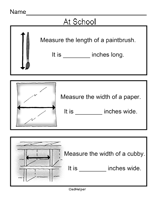I Can Measure It Myself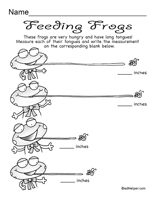Ruler Puzzles

Measurement Logic Puzzles
Width and length of rooms (feet)
Width and length of vegetable plots (meters and centimeters)
Length, width, height, and volume (same unit)
Length, width, height, and volume (mix of units)

Measurement
Metric units of length

English Measurement
English Length

Metric System: Metric System Worksheets
Metric Length

Metric System: Metric System Crossword Number Puzzles
Metric Length

Perimeter

Measurement

Have a suggestion or would like to leave feedback?